WO2012008751A2 - Method and apparatus for sensing a plurality of contact inputs - Google Patents

Method and apparatus for sensing a plurality of contact inputs Download PDF

Info

Publication number
WO2012008751A2
WO2012008751A2 PCT/KR2011/005153 KR2011005153W WO2012008751A2 WO 2012008751 A2 WO2012008751 A2 WO 2012008751A2 KR 2011005153 W KR2011005153 W KR 2011005153W WO 2012008751 A2 WO2012008751 A2 WO 2012008751A2
Authority
WO
WIPO (PCT)
Prior art keywords
sensing
step
input
signal
contact
Prior art date
Application number
PCT/KR2011/005153
Other languages
French (fr)
Korean (ko)
Other versions
WO2012008751A3 (en
Inventor
남성식
Original Assignee
(주)멜파스
Priority date (The priority date is an assumption and is not a legal conclusion. Google has not performed a legal analysis and makes no representation as to the accuracy of the date listed.)
Filing date
Publication date
Priority to KR1020100069075A priority Critical patent/KR101696386B1/en
Priority to KR10-2010-0069075 priority
Application filed by (주)멜파스 filed Critical (주)멜파스
Publication of WO2012008751A2 publication Critical patent/WO2012008751A2/en
Publication of WO2012008751A3 publication Critical patent/WO2012008751A3/en

• 230000027455 binding Effects 0 description 1
• 238000009739 binding Methods 0 description 1
• 238000004364 calculation methods Methods 0 description 3
• 239000003990 capacitor Substances 0 description 16
• 230000003197 catalytic Effects 0 description 1
• 238000007600 charging Methods 0 claims description 6
• 230000001276 controlling effects Effects 0 claims description 7
• 238000007796 conventional methods Methods 0 description 1
• 230000000875 corresponding Effects 0 description 2
• 238000001514 detection method Methods 0 claims description 23
• 239000010432 diamond Substances 0 description 1
• 238000009826 distribution Methods 0 description 1
• 230000001747 exhibited Effects 0 description 2
• 230000001965 increased Effects 0 description 2
• 230000015654 memory Effects 0 description 1
• 238000000034 methods Methods 0 description 2
• 238000006011 modification Methods 0 description 1
• 230000004048 modification Effects 0 description 1
• 230000003287 optical Effects 0 description 1
• 230000001603 reducing Effects 0 description 1
• 230000004044 response Effects 0 claims description 6
• 230000035945 sensitivity Effects 0 description 3
• 230000001360 synchronised Effects 0 description 1

Images

•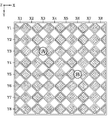•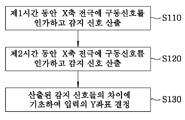•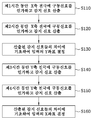•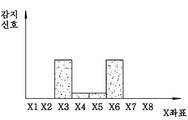•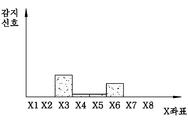•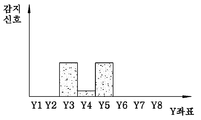•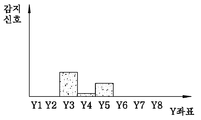•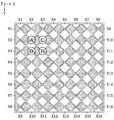•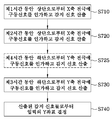•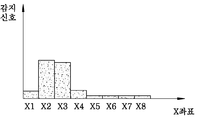•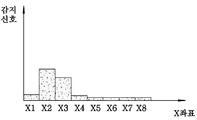•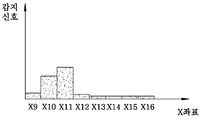•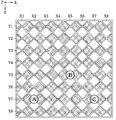•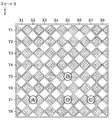•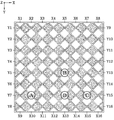•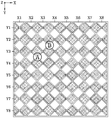•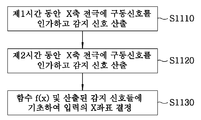•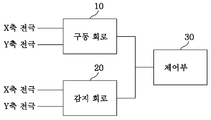Classifications

• GPHYSICS
• G06COMPUTING; CALCULATING; COUNTING
• G06FELECTRIC DIGITAL DATA PROCESSING
• G06F3/00Input arrangements for transferring data to be processed into a form capable of being handled by the computer; Output arrangements for transferring data from processing unit to output unit, e.g. interface arrangements
• G06F3/01Input arrangements or combined input and output arrangements for interaction between user and computer
• G06F3/03Arrangements for converting the position or the displacement of a member into a coded form
• G06F3/041Digitisers, e.g. for touch screens or touch pads, characterised by the transducing means
• G06F3/044Digitisers, e.g. for touch screens or touch pads, characterised by the transducing means by capacitive means
• GPHYSICS
• G06COMPUTING; CALCULATING; COUNTING
• G06FELECTRIC DIGITAL DATA PROCESSING
• G06F2203/00Indexing scheme relating to G06F3/00 - G06F3/048
• G06F2203/041Indexing scheme relating to G06F3/041 - G06F3/045
• G06F2203/04104Multi-touch detection in digitiser, i.e. details about the simultaneous detection of a plurality of touching locations, e.g. multiple fingers or pen and finger

Abstract

The present invention relates to a method for sensing contact inputs in a contact input sensing apparatus comprising a sensing electrode. The method for sensing contact inputs comprises: a first step of enabling an input terminal of the sensing electrode to supply a driving signal for a first time period so as to obtain a sensed signal; a second step of enabling the input terminal of the sensing electrode to supply a driving signal for a second time period so as to obtain a sensed signal; and a third step of determining the location of the contact input based on the difference between the sensed signal obtained in the first step and the sensed signal obtained in the second step, wherein the first time period and the second time period are different from each other. The coordinates of the contact inputs can thereby be accurately determined even when two or more contact inputs are applied.

Description

Method and apparatus for detecting a plurality of contact inputs

The present invention relates to a method and apparatus for sensing a touch input of a display device, and more particularly, to a method and an apparatus for accurately determining a position of a touch input when there are two or more touch inputs.

The touch sensing device detects a touch of a user's finger or another device and converts the touch into a suitable electric signal and outputs the same. For example, it is applied to a laptop computer and used as an input means for controlling the movement of a cursor by replacing a mouse, or as an input means for directly selecting and executing an icon or a menu displayed on a screen in combination with a display device. . It is simply used as a means of replacing buttons. In recent years, as the screen of an electronic device becomes larger and the device becomes smaller, a touch input device (for example, a touch screen) combined with a display is excluded from an input device such as a keypad, and the only input means (at least as a main input). Increasingly, the use) is increasing.

As the application of the touch sensing device is expanded, a device that recognizes two or more touch inputs at the same time and performs a promised operation according to its position has been introduced. For example, it is possible to recognize two or more contact inputs at the same time so that one input controls the position of the cursor and the click input is implemented by the other input. In addition, a method of rotating a screen by moving one input based on one input or a method of enlarging or reducing the screen according to a change in distance between two inputs may be implemented.

In order to receive two or more contact inputs and to use them, it is essential to accurately determine the location of the contact input. However, in the prior art, the position of two or more contact inputs could not be clearly determined. For example, if there are two contact inputs, the conventional method provides two X coordinates of the position where the contact input is considered to exist and two Y coordinates of the position where the contact input is considered to be present, respectively. Therefore, there are 4 (that is, 2 x 2) branches in the case of the coordinates (X, Y) of the touch input, and it was not possible to determine which of them is the coordinate of the true touch input. In addition, when the position of the touch input is sensed using a sensing electrode disposed discontinuously, when two or more touch inputs are applied to one sensing electrode, it is difficult to accurately determine the position of the input.

It is therefore an object of the present invention to provide a touch input sensing method and apparatus capable of clearly determining the coordinates of each touch input when there are two or more touch inputs.

In order to achieve the above object, according to an aspect of the present invention, in a method for sensing a touch input in a touch input sensing device including a sensing electrode, supplying a drive signal from the input terminal of the sensing electrode for a first time; A first step of calculating a sensing signal for the touch input, a second step of supplying a driving signal from the input terminal of the sensing electrode for a second time and calculating a sensing signal for the touch input, and the first step And determining a location of the touch input based on a difference between the detected signal calculated in step 2 and the detected signal calculated in the second step, wherein the first time and the second time are different from each other. A method is provided.

Preferably, the sensing electrode has an input terminal formed at two ends facing each other, and the second step includes supplying a driving signal to each of the two input terminals to calculate a sensing signal.

The touch input sensing device includes two or more first sensing electrodes extending in a first direction, wherein the first and second steps are performed for each of the first sensing electrodes, and the third step is the contact. Preferably, the position of the first direction of the input is determined.

Preferably, in the third step, the driving signal is supplied to the first direction position of the contact input with respect to a sensing electrode having a larger difference between the sensing signals calculated in the first and second steps. Determine further away from the input terminal of the first electrode.

The touch input sensing device includes two or more second sensing electrodes extending in a second direction crossing the first direction, the method comprising: supplying a drive signal to each of the second sensing electrodes for a third time period and A fourth step of calculating a sensing signal for a touch input, a fifth step of supplying a driving signal from an input terminal of each of the second sensing electrodes for a fourth time, and calculating a sensing signal for the touch input, and the fourth And a sixth step of determining a position of the second direction of the touch input based on a difference between the detection signal calculated in the step and the detection signal calculated in the fifth step, wherein the third time and the fourth time are May differ from one another.

The second sensing electrode may have input terminals formed at two ends facing each other, and the fifth step may include calculating driving signals by supplying driving signals to the two input terminals, respectively.

In the sixth step, the second electrode is supplied with the driving signal to the second direction position of the contact input with respect to the sensing electrode having a larger difference between the sensing signals calculated in the fourth and fifth steps. It is desirable to determine further away from the input.

In addition, the first step or the second step includes calculating at least two second direction positions of the touch input from the detection signal, wherein the sixth step is one of the two or more calculated second direction positions. It is preferable to include the step of selecting.

The fourth step or the fifth step includes calculating at least two first direction positions of the touch input from the sensing signal, and the third step selects one of the two or more calculated first direction positions. It is also preferable to include.

There may be three or more contact inputs.

Preferably, the first step includes determining whether there are two or more touch inputs from the sensing signal, and wherein the second step is determined when there are two or more touch sensing electrodes in the first step. Is performed.

Also, preferably, the fourth step may include determining whether there are two or more touch inputs from the sensing signal, and the fifth step may determine that there are two or more touch sensing electrodes in the fourth step. Is performed in case.

Advantageously, said third step determines the position of said contact input further based on a known relationship between the distance from said input and said sensing signal.

The driving signal is applied to be transmitted in a first direction, the contact input is two or more, and the third step includes: determining the position of the second direction crossing the first direction of the contact input; It is also desirable to include calculating an area ratio between the contact inputs further based on the distance from to each of the contact inputs.

The step of calculating the area ratio is expressed by equation

And The area ratio between the contact inputs may be calculated based on Equation 1 , wherein I 1 is a sensing signal calculated in the first step, I 2 is a sensing signal calculated in the second step, and R is a contact input at the input terminal. The sensing signal when applied, f 1 (x) is the sensing signal at the x position from the input terminal when the first drive signal is applied, f 2 (x) is the sensing signal at the x position from the input terminal when the second driving signal is applied , S a and S b represent the sensitivity of the sensing signal by the touch input, and a and b represent the distance from the input terminal to the respective touch inputs.

The driving signal may include a charge, and the sensing signal may be based on a capacitance of the sensing electrode.

In addition, a shorter time of the first time and the second time may be a time for partially charging the capacitance formed in the sensing electrode, and a shorter time of the third time and the fourth time is formed in the sensing electrode. It may be time to partially charge the capacitance.

The method may further include determining the number of touch inputs based on the strength of the sensing signal.

According to another aspect of the present invention, there is provided a sensing electrode, a driving circuit for applying a driving signal to the sensing electrode, a sensing circuit for calculating a sensing signal for a touch input in response to the driving signal, and the driving circuit and the sensing circuit. A touch input sensing device comprising a controller for controlling, wherein the controller is provided with a touch input sensing device for controlling the driving circuit and the sensing circuit to perform the above-described method.

According to yet another aspect of the present invention, in a controller capable of controlling a driving circuit to apply a driving signal to a sensing electrode, and controlling the sensing circuit to produce a monitoring signal in response to the driving signal, wherein the controller is A controller is provided which controls the drive circuit and the sense circuit to perform the method as claimed in claim 1.

Preferably, at least one of the driving circuit and the sensing circuit is included in the controller.

According to the present invention, even when there are two or more contact inputs, the coordinates of each contact input can be clearly determined.

1 illustrates a touch input sensing device in accordance with an embodiment of the present invention.

2 is a flowchart for explaining a touch input sensing method according to an embodiment of the present invention.

3 is a flowchart for explaining a touch input sensing method according to another embodiment of the present invention.

4A and 4B show sensing signals measured for an X-axis electrode, respectively, in accordance with one embodiment of the present invention.

5A and 5B show sense signals measured for a Y-axis electrode, respectively, in accordance with one embodiment of the present invention.

6 illustrates a touch input sensing device according to another embodiment of the present invention.

7 is a flowchart for explaining a touch input sensing method according to another embodiment of the present invention.

8A-8C illustrate sensing signals measured for an X-axis electrode in accordance with one embodiment of the present invention.

9A to 9C are views showing a state of a touch sensing device to which three or more inputs are applied.

10 is a view of a touch input sensing device for explaining a touch input sensing method according to another embodiment of the present invention.

11 is a flowchart for explaining a touch input sensing method according to another embodiment of the present invention.

12 is a diagram illustrating a configuration of a touch sensing apparatus according to an embodiment of the present invention.

Hereinafter, specific embodiments of the present invention will be described with reference to the accompanying drawings.

1 is a diagram illustrating a touch sensing device used in a display device according to an embodiment of the present invention. The touch sensing apparatus of the present embodiment includes a plurality of, for example, eight X-axis sensing electrodes X1 to X8 and a plurality of, for example, eight Y-axis sensing electrodes Y1 to Y8. The axis sensing electrodes X1-X8 extend in the Y axis, and the Y axis sensing electrodes Y1-Y8 extend in the X axis. In this embodiment, each of the sensing electrodes has a shape in which a plurality of rhombic electrodes are connected to each other so as to cover the entire area of the touch sensing device while minimizing the overlapping area. However, the shape of the sensing electrodes is not limited thereto. The X-axis sensing electrodes X1-X8 and the Y-axis sensing electrodes Y1-Y8 may be respectively connected to the driving circuit 10 and the sensing circuit 12 of FIG. 12, for example. The driving circuit and the sensing circuit may be implemented in one circuit.

In a capacitive touch sensing device, when a touch input is applied to a capacitor formed by an electrode, a change in capacitance occurs by contact, and the presence of the touch input on the touch screen is measured by measuring the change. Will be detected. As one of the methods for sensing the change in capacitance, there is a method of measuring the voltage value of the electrode while continuously supplying a driving signal, for example, a charge to the electrode. In general, when a constant current is supplied, the relationship between the capacitance and the voltage may be expressed by Equation 1 below.

Equation 1

Where t is time, C is capacitance, v is voltage and i is current.

As can be seen from the above equation, if the current is applied for the same time, the larger the electrode, the lower the voltage.

Therefore, in the touch sensing device of the present embodiment, the driving circuit applies electric charge as a driving signal to each of the electrodes X1-X8 and Y1-Y8, and the sensing circuit measures the voltage generated at each electrode according to the application of the electric charge. The capacitance at the electrode can be determined, and a signal representing the measured capacitance can be used as a detection signal representing the application of the contact input.

In the present embodiment, a capacitive touch sensing device is described, but the present invention is not limited to the capacitive touch sensor, and the present invention can be applied to a touch sensing device using various parameters such as pressure, temperature, resistance, and optical characteristics. . In this case, a signal representing the value of each parameter can be used as the detection signal.

As used herein, the term "sense signal" refers to a signal used to indicate the strength of a touch input, and is described as the intensity of the sense signal is proportional to the touch input. The contact input generates a contact region between the sensing electrode and the contact object, and a capacitance change occurs in the sensing electrode according to the size of the contact region. Thus, the intensity of the sense signal can be used to calculate how wide a contact area the contact input forms with a particular sense electrode. However, it should be noted that the actual implementation may use a sense signal that is inversely proportional to the strength of the touch input, and any signal that directly or indirectly represents the strength of the touch input is included in the sense signal.

Hereinafter, with reference to FIG. 2 illustrating a touch input sensing method according to an embodiment of the present invention, two touch inputs to coordinates (X3, Y3) and (X6, Y5) in the touch sensing device of the embodiment of FIG. A method of detecting contact input when A and B are generated will be described.

First, in step S110, a charge is supplied to each of the X-axis sensing electrodes X1-X8 for a predetermined first time, and the strength of the sensing signal that senses a touch input is calculated. As described above, the sense signal can be calculated by measuring the capacitance at each electrode. Next, in step S120, charges are supplied to each of the X-axis sensing electrodes X1-X8 for a predetermined second time, and the strength of the sensing signal sensing the touch input is calculated. Next, in step S130, a sensing electrode having a touch input among Y electrodes is determined based on a difference between the strength of the sensing signal calculated in step S110 and the strength of the sensing signal calculated in step S120.

Here, the first time and the second time may be determined differently. For example, the first time may be sufficient time for the capacitor formed in the sensing electrode to be fully charged, while the second time may be shorter than the first time. In another embodiment, the second time is a time for partially charging the capacitance formed in the sensing electrode, and the first time may be a time longer than the second time. In another embodiment, the first time may be shorter than the second time.

This difference in time results in a difference in the degree of charge of the capacitor depending on the position. For example, the X-axis sensing electrodes X1-X8 are connected to the driving circuit (for example, the driving circuit 10 of FIG. 12) at the upper end thereof in the embodiment of FIG. 1. Thus, the electric charge applied from the driving circuit is transferred from the upper end (ie, the end connected to the driving circuit) to the lower end (ie, the opposite end of the end connected to the driving circuit) through the electrodes X1-X8, and in the process Pass the resistance by (X1-X8). In the simplified model, the circuit's time constant is

= R · C (where R is the resistance and C is the capacitance) and is proportional to the resistance, so the more the resistor passes, the longer the time required to charge the capacitor.

Therefore, the charging time of the capacitor formed close to the driving circuit (i.e., the capacitor with which the relatively small resistance is connected) is shorter than the charging time of the capacitor formed (ie, the capacitor with the relatively large resistance) connected from the driving circuit.

When the charge is supplied to the sensing electrode only for a short time using this characteristic, the sensing electrode farther from the driving circuit shows a lower voltage, and accordingly, it is determined that the sensing electrode exhibits a low capacitance, that is, a weak sensing signal. . On the other hand, if the charge is supplied for a sufficient time, all the capacitors can be fully charged, so the difference in the sense signal is not large.

For example, when the charge is supplied for a first time, which is a time sufficient for the capacitor to be charged, the sense signal measured at the X-axis electrodes indicates the position of the electrode where the contact input is present (ie, as shown in FIG. 4A). Coordinates), X3 and X6 are not significantly different. On the other hand, when charge is supplied for a second time which is shorter than the first time, as shown in FIG. 4B, a large contact input is detected at X3 where the contact input A, which is a contact input close to the upper end to which the driving circuit is connected, is located. On the other hand, relatively small contact inputs are detected at X6.

That is, the difference between the touch inputs sensed in steps S110 and S120 is greater at X6 far from the drive than in X3 close to the drive. Therefore, in step S130, on the basis of the difference in the degree of contact input calculated in steps S110 and S120, the contact input B at the electrode having the large difference (i.e., X6) is far from the driving circuit, i.e., Y It can be determined that the coordinates are large. Therefore, even if two X coordinates X3 and X6 and two Y coordinates Y3 and Y5 are given, it can be determined that the Y coordinate of the contact input at X6 is larger. In other words, in step S130, for the sensing electrode having a larger difference between the sensing signals calculated in steps S110 and S120, the Y direction position or coordinate of the contact input may be determined farther from the charge input terminal of the X-axis electrode. . This makes it possible to uniquely determine two contact inputs (i.e., input A of (X3, Y3) and input B of (X6, Y5)).

In one embodiment, in step S110 it is determined whether there are two or more touch inputs from the calculated sense signal, and step S120 may be performed when it is determined in step S110 that there are two or more touch sense electrodes. In step S110, a charge is supplied to each of the X-axis electrodes X1-X8 and a sensing signal is calculated, and it is determined therefrom that the position where there is a contact input is two or more. In one embodiment, it may be determined that there is a contact input at that location when the sense signal exceeds a predetermined threshold. If the coordinates of the contact input cannot be determined uniquely, it is limited to the case where both the X coordinate and the Y coordinate of the determined contact input are two or more, and if only one is one, the coordinates of the contact input can be determined. The calculation amount and the operation time can be reduced by performing step S120 only when the contact input is determined to be 2 or more in S110.

Meanwhile, the above steps S110 to S130 may be additionally performed on the Y-axis sensing electrodes Y1-Y8, and are not performed on the X-axis sensing electrodes X1-X8, but not on the Y-axis sensing electrodes Y1-Y8. It may also be performed for. When the steps are additionally performed for the Y-axis sensing electrodes Y1-Y8, as shown in FIG. 3, in step S140, charges are supplied to each of the Y-axis sensing electrodes Y1-Y8 for a third time, and the sensing signal is detected. Calculate In operation S150, charge is supplied from the charge input terminal of each of the Y-axis sensing electrodes Y1-Y8 for the fourth time, and the sensing signal is calculated. In operation S150, the X-axis position (that is, coordinates) of the contact input is determined based on the difference between the detection signal calculated in step S130 and the detection signal calculated in step S140.

Here, the third time and the fourth time may be determined differently. For example, the third time may be sufficient time for the capacitor formed in the sensing electrode to be fully charged, while the fourth time may be shorter than the third time. In another embodiment, the fourth time may be a time for partially charging the capacitance formed in the sensing electrode, and the third time may be longer than the fourth time. In another embodiment, the third time may be shorter than the fourth time.

By varying the charge supply time in this way, the coordinates of the contact input can be determined uniquely. For example, when the contact inputs A and B shown in FIG. 1 are applied, when the charge is supplied for a third time, which is a time sufficient for the capacitor to be charged, the sensing signal measured at the Y-axis electrodes is shown in FIG. As shown in 5a, there is no significant difference in Y3 and Y5, the coordinates at which the contact input is present. On the other hand, when charge is supplied for a fourth time, which is shorter than the third time, as shown in FIG. 5B, a large contact input is detected at Y3 where the contact input A, which is a close contact input from the left side to which the driving circuit is connected, is located. In contrast, relatively small contact inputs are detected at Y5. Therefore, it can be determined that the contact input having a large change in the sense signal is far from the driving circuit connection end, and finally it can be determined that the X coordinate of the contact input corresponding to Y5 is X5 far from the left.

In FIG. 3, another embodiment in which the sensing signal is calculated twice for both the X-axis and Y-axis sensing electrodes has been described. However, substantially, if there are two contact inputs, the coordinates of the contact inputs can be determined even if two sensing signals are calculated for only one of the X-axis sensing electrode and the Y-axis sensing electrode. For example, one detection signal is calculated for the Y-axis electrode to obtain two Y-axis coordinates, and two detection signals are obtained for the X-axis electrode to obtain two X-axis coordinates. By determining whether the Y coordinate of the sense signal corresponding to the coordinate is larger, it is possible to determine the exact coordinate of the contact input. Thus, in actual implementation, not all steps shown in FIG. 2 need to be performed. In addition, the steps need not be executed in the same order as shown in FIG. Depending on the implementation, it is also possible to drive the X and Y axes electrodes alternately or simultaneously.

6 is a diagram illustrating a touch input sensing device according to another embodiment of the present invention. The touch sensing device of the present embodiment is substantially the same as the apparatus of FIG. 1 except that each sensing electrode is connected to the driving circuit at both ends, for example, at its upper and lower ends in FIG. 6. In the sensing electrode of the present embodiment, separate coordinates are provided to each connection point at both ends. For example, in the embodiment of Fig. 6, the upper connection point is given the coordinates of X1 to X8 for the X-axis sensing electrode, while the lower connection point is given the coordinates of X9 to X16. Further, the coordinates of Y1 to Y8 are given to the connection point on the left side to the Y-axis sensing electrode, while the coordinates of Y9 to Y16 are given to the connection point on the right side. However, the method of assigning the coordinates is not limited to the illustrated ones, and if a separate monitoring signal can be obtained at both ends of each sensing electrode and can be distinguished from them, the coordinates may be provided in any manner. It is also possible to distinguish the sensing electrodes in other ways.

Hereinafter, with reference to FIG. 7 illustrating a touch input sensing method according to an embodiment of the present invention, coordinates (X2, Y2) and (with reference to upper and left coordinates) of the touch sensing device of the embodiment of FIG. The touch input sensing method in the case where two touch inputs A and B are input to X3, Y3) will be described. In the following description, when driving the X-axis sensing electrode at the upper end, each sensing electrode is identified by the coordinates of X1-X8, and when driving at the lower end, it is identified by the coordinates of X9-X16. Similarly, when driving the Y-axis sensing electrode on the left side, each sensing electrode is identified by the coordinates of Y1-Y8, and when driving on the right side, it is identified by the coordinates of Y9-Y16.

First, a charge is supplied to each of the X-axis sensing electrodes X1-X8 for the first predetermined time in step S710, and the intensity of the sensing signal is calculated. Next, in step S720, charges are supplied to each of the X-axis sensing electrodes X1 to X8 for a second predetermined time on the same side as S710, and the strength of the sensing signal is calculated. Here, the first time and the second time may be different from each other as in the previous embodiment. Again, in step S730, a charge is supplied to each of the X-axis sensing electrodes X9-X16 for a predetermined third time at the side different from step S710 to calculate the intensity of the sensing signal. Again, the third time and the first time may be different times from each other. Finally, Y is based on the difference between the strength of the sensing signal calculated in step S710 and the strength of the sensing signal calculated in step S720 and the difference between the strength of the sensing signal calculated in step S710 and the strength of the sensing signal calculated in step S730. The sensing electrode in which the touch input exists among the electrodes is determined (step S740). As described above, in the embodiment of FIG. 7, the detection signal is repeatedly calculated with different driving directions for the same electrode. Therefore, unlike the embodiment of Fig. 2, two pairs of data for determining the position of the contact input can be obtained, and the accuracy of the contact input position calculation can be improved.

For example, when two contact inputs are applied to positions A and B as shown in FIG. 6, the sensing signals calculated in steps S710 and S720 are as shown in FIGS. 8A and 8B, respectively. 8A and 8B illustrate the case where the first time is sufficient time to fully charge the capacitor of the sensing electrode, and the second time is shorter than the first time. As can be seen in FIG. 8B, since the sensing inputs are arranged adjacent to each other, the difference in the intensity of the sensing signal at the coordinates X2 and X3 is not large. Therefore, it is difficult to reliably identify which of the contact inputs in X2 and X3 is close to the top only by the difference of the signals measured in steps S710 and S720, and determine whether a given input is A and B or C and D. Is difficult. However, when driving the electrode from the other direction in step S730 to obtain the detection signal intensity distribution as shown in Figure 8c, it is possible to obtain the detection signal difference between Figure 8a and 8b and the detection signal difference between Figure 8a and 8c, these information Can be combined to determine the Y coordinate of the contact input more precisely.

Here, as illustrated by a dotted line, a signal comparable with the sensing signal obtained in step S730 may be obtained, including step S725 of driving the sensing electrode in the same direction as step S730 before step S730. That is, it is also possible to drive the sensing electrodes twice in different directions for different times. However, if the sensing electrode is driven for a time sufficient to charge the capacitor of the sensing electrode in step S725, substantially the same sensing signal as in step S710 will be obtained, so in steps S725 and S725, the capacitors of the sensing electrode are completely removed. It would be desirable if it was driven for a period of time that could not be charged.

In one embodiment, in step S710 it is determined whether there are two or more touch inputs from the calculated sensing signal, and steps S720 and S730 can be performed only when it is determined in step S710 that there are two or more touch sensing electrodes. In step S710, electric charges are supplied to each of the X-axis electrodes X1-X8, and a sensing signal is calculated, from which it is determined whether the position at which there is a contact input is two or more. In one embodiment, it may be determined that there is a contact input at that location when the sense signal exceeds a predetermined threshold. Performing steps S720 and S730 only when the contact input is determined to be 2 or more in step S710 can reduce the amount of calculation and the operation time.

Further, in another embodiment, steps S710 to S740 may be additionally performed for the Y-axis sensing electrode, or may be performed only for the Y-axis sensing electrode and not for the X-axis sensing electrode. That is, first, the driving is performed for the sensing electrodes Y1-Y8 for a first time, and the driving for the sensing electrodes Y1-Y8 is performed for a second time different from the first time. Further, after the driving is performed for the sensing electrodes Y9-Y16 for a third time different from the first time, the X coordinate of the contact input is determined using the sensing signal obtained by three drivings. For example, in the example of FIG. 3, it is possible to determine that the contact input of A and B, rather than C and D, is applied by grasping that the sensing input is applied near the left side in Y2.

On the other hand, the method of the present invention can be used to determine the exact position of the contact input even when there are three or more contact inputs. That is, three coordinate pairs can be determined by obtaining three coordinates for each of the X-axis and the Y-axis, and also grasping the relative position of the sensing input at each coordinate based on the difference of the sensed signals calculated twice. However, when two or more contact inputs have the same X or Y coordinate, additional processing may be required, which will be described with reference to FIGS. 9A to 9C.

9A shows a touch input sensing device to which three touch inputs are applied, and 9b and 9c show a touch input sensing device to which four touch inputs are applied.

First, as shown in FIG. 9A, when three contact inputs A, B, and C are applied, driving the sensing electrode once causes three X coordinates (X2, X5, X7) and two Y coordinates (Y5, Y7) can be obtained. On the other hand, if the difference in the detection signal is calculated through the second drive with respect to the X-axis sensing electrode, it can be seen that the input B in X5 is relatively close to the upper end, and thus the Y of the input B in X5. It can be determined that the coordinate is Y5.

Next, as shown in FIG. 9B, four contact inputs A, B, C, and D are applied, but the three inputs A, D, and C have the same Y coordinate and two inputs B and D. Consider the case of having the same X coordinate. In this case, three X coordinates (X2, X5, X7) are obtained through one drive on the X-axis sensing electrode, and two Y coordinates (Y5, Y7) are obtained through one drive on the Y-axis sensing electrode. . In addition, through the second drive to the X-axis sensing electrode, it can be seen that the contact input at X5 is relatively close to the upper end. However, through the above process, the coordinates calculated for the contact inputs of FIG. 9B and the coordinates calculated for the contact inputs of FIG. 9A are substantially the same. That is, the contact input D may be missing. Thus, in one embodiment, the number of contact inputs can be determined based on the strength of the sense signal at each sense electrode. For example, in order to grasp the characteristics of the contact input of FIG. 9B, the intensity of the detection signal measured at the Y7 position is stronger than that of the contact input shown in FIG. 9A through the second driving on the Y axis. Figure out. This makes it possible to grasp that the Y7 electrode has three contact inputs, and determine the coordinates of the four contact inputs based on the information.

In another embodiment, four contact inputs A, B, C, and D may be recognized using sense electrodes connected at both ends to the drive circuit as shown in FIG. 9C. Specifically, first, three X coordinates (X2, X5, X7) are obtained through one drive on the X-axis sensing electrode, and two Y coordinates (Y5, Y7) through one drive on the Y-axis sensing electrode. Get In addition, the second drive from the upper end with respect to the X-axis sensing electrode can be seen that the contact input at X5 is relatively close from the upper end. In addition, when the third drive from the bottom to the X-axis sensing electrode, it can be seen that the contact input at X13 is relatively close to the bottom. Therefore, it can be seen that there are two inputs at the position X5 (or X13), and the coordinates of the four contact inputs can be determined without missing the contact input of D. Although the case where three contact inputs having the same Y coordinate are applied has been described, the above embodiment may be equally applied even when three contact inputs having the same X coordinate are applied, but in this case, the Y-axis sensing electrode The only difference is that the drive is made three times for.

Further, according to another embodiment of the present invention, by applying the above-described principle, there is provided a method for determining the exact position of these contact inputs when two or more contact inputs are applied to one sensing electrode, which is illustrated in FIGS. 10 and FIG. It demonstrates with reference to 11.

Referring to FIG. 10, two contact inputs A and B are applied to one sensing electrode X3. Since these contact inputs are only partially applied to the sensing electrode X3, the position of the contact input (i.e., the position of the center of the touch input) should be determined as the position between X3 and the adjacent sensing electrode, not the sensing electrode X3. Specifically, since contact input A is applied to both sensing electrodes X2 and X3 and contact input B is applied to both sensing electrodes X3 and X4, the position of contact input A is between sensing electrodes X2 and X3 and the position of contact input B. Is between the sensing electrodes X3 and X4. Thus, to accurately determine the location of each contact input, it must be possible to determine what percentage of the contact inputs were applied to each electrode. However, in the sensing electrode X3, only the sensing signals generated by the contact inputs A and B overlap and are sensed, and it is difficult to determine the strength of each sensing signal generated by each touch input.

The present embodiment calculates the intensity of the sense signal by each contact input by driving the sense electrode twice for different time to calculate the sense signal. Referring to Fig. 11, first, the method of the present embodiment applies a driving signal to the sensing electrode X3 for the first time and calculates the sensing signal (step S1110). In addition, a driving signal is applied to the sensing electrode X3 for the second time and the sensing signal is calculated (step S1120).

On the other hand, the sensing signal at the distance x from the charge input terminal when the driving signal is applied to the sensing electrode X3 for the first time is f 1 (x), and the charge when the driving signal is applied to the sensing electrode for the second time. It is assumed that the sense signal at distance x from the input stage is given by f 2 (x). This function f (x) is, as described above, due to the difference in the magnitude of the resistance passing as the driving signal is transmitted on the sensing electrode, the time constant changes according to the position on the electrode, and the magnitude of the sensing signal measured accordingly. Is a function representing the relation to be changed and may be calculated mathematically or determined through experiments. The function f (x) may be stored in advance in, for example, an internal memory of the touch sensor chip, and used to calculate the contact position.

Then, in step S1130 it is possible to determine the strength of the detection signal generated by each contact input based on the known function f (x) and the detection signals measured in the first and second steps. Specifically, since the sensing signal I 1 calculated in the first step is the sum of the sensing signal by the touch input A and the sensing signal for the touch input B, it may be given by Equation 2 below.

Equation 2

Here, S a and S b represent the sensitivity of the detection signal by the contact inputs A and B, respectively, and are values proportional to the contact area. On the other hand, a and b are values representing distances or Y coordinates from the charge input terminals of the contact inputs A and B, respectively, and may be determined in various ways. For example, a and b may be determined by detecting a sensing signal at the Y-axis sensing electrode.

Similarly, the sensing signal I 2 calculated in the second step may be given by the following equation (3).

Equation 3

Solving the equations (2) and (3) together, the sensitivity S a and S b by the respective contact inputs can be obtained, and accordingly, it can be seen how much sensed signal each contact input generated. As a result, by detecting the strength of the detection signal generated by the touch input at the sensing electrode, it is possible to determine the exact position of each detection signal. The position determined here is a position in a direction intersecting with the extending direction of the sensing electrode (that is, the Y axis direction), for example, the X coordinate of the contact input.

The methods described above may be performed by a driving circuit and / or a sensing circuit. An example of the configuration of the touch input sensing device is shown in FIG. 12. The driving circuit 10 is connected to the X-axis sensing electrode and / or Y-axis sensing electrode, and applies a driving signal to the electrode for a predetermined time as needed. Here, although one driving circuit is illustrated, the driving circuit 10 may include two driving circuits respectively connected to both ends of the electrode. Alternatively, one driving circuit may be connected to both ends of the electrode to perform separate driving from both ends of the electrode. In addition, the sensing circuit 20 is connected to the X-axis sensing electrode and the Y-axis sensing electrode, and calculates a sensing signal for each electrode in response to the driving signal applied from the driving circuit 10. The driving circuit 10 and the sensing circuit 20 are controlled by the controller 30 so that the application of the driving signal and the calculation of the sensing signal can be synchronized. These driving circuits and sensing circuits may be separate circuits or may be integrated circuits. Each drive circuit and sense circuit may include one or more modules for performing the above methods, which may be implemented as software modules, hardware modules, or a combination thereof. Such drive and sense circuits may be operable to perform the method under the control of a controller. Meanwhile, one or both of the above-described driving circuit and sensing circuit may be included in the controller. In this case, it is also possible for the controller to be configured in the form of an integrated circuit.

In addition, the methods may be implemented in the form of a program, and may be performed by a computer.

The present invention has been described above with reference to specific embodiments of the present invention, but this is only illustrative and does not limit the scope of the present invention. Those skilled in the art can change or modify the described embodiments without departing from the scope of the present invention. Each of the functional blocks or means described herein may be implemented by various well-known elements such as an electronic circuit, an integrated circuit, an application specific integrated circuit (ASIC), and the like. Can be. Components such as means described as separate in the specification and claims may be simply functionally distinct and may be physically implemented as one means, and components such as means described as a single element may be It can be made in combination. In addition, each method step described herein may be changed in order without departing from the scope of the present invention, and other steps may be added. In addition, the various embodiments described herein may be implemented independently as well as each other as appropriate. Therefore, the scope of the invention should be defined by the appended claims and their equivalents, rather than by the described embodiments.

Claims (19)

1. A method of sensing a touch input in a touch input sensing device comprising a sensing electrode,
A first step of supplying a driving signal from an input terminal of the sensing electrode for a first time and calculating a sensing signal for the contact input;
Supplying a driving signal from the input terminal of the sensing electrode for a second time and calculating a sensing signal for the contact input;
And a third step of determining a position of the contact input based on a difference between the detection signal calculated in the first step and the detection signal calculated in the second step.
Wherein the first time and the second time are different.
2. The method of claim 1,
The sensing electrode has input terminals formed at two ends facing each other,
The second step includes supplying driving signals to two input terminals, respectively, to calculate a detection signal.
3. The method of claim 1,
The touch input sensing device includes two or more first sensing electrodes extending in a first direction,
The first step and the second step are performed for each of the first sensing electrodes,
And wherein said third step determines the position of said first direction of said contact input.
4. The method of claim 1,
In the third step, the sensing electrode having a larger difference between the detection signals calculated in the first step and the second step is set to the first direction position of the contact input of the first electrode to which the driving signal is supplied. Contact input detection method to determine further away from the input.
5. The method of claim 1,
The touch input sensing device includes two or more second sensing electrodes extending in a second direction crossing the first direction,
The method,
Supplying a driving signal to each of the second sensing electrodes for three hours and calculating a sensing signal for the contact input;
A fifth step of supplying a driving signal from an input terminal of each of the second sensing electrodes for 4 hours and calculating a sensing signal for the touch input;
And a sixth step of determining the position of the second direction of the touch input based on the difference between the detection signal calculated in the fourth step and the detection signal calculated in the fifth step.
And the third time and the fourth time are different from each other.
6. The method of claim 5,
The second sensing electrode has input terminals formed at two end portions facing each other,
The fifth step includes supplying driving signals to two input terminals, respectively, and calculating a sensing signal for the touch input.
7. The method of claim 5,
In the sixth step, the second electrode is supplied with the driving signal to the second direction position of the contact input with respect to the sensing electrode having a larger difference between the sensing signals calculated in the fourth and fifth steps. Contact input detection method to determine further away from the input.
8. The method of claim 5,
Wherein said first step or said second step includes calculating at least two second direction positions of a touch input from said sensing signal;
And the sixth step includes selecting one of the two or more calculated second directional positions.
9. The method of claim 5,
The fourth step or the fifth step includes calculating at least two first direction positions of the touch input from the detection signal,
And wherein said third step includes selecting one of said two or more calculated first directional positions.
10. The method of claim 3, wherein
The first step includes determining whether there are two or more contact inputs from the sense signal,
And wherein the second step is performed when it is determined in the first step that there are two or more touch sensing electrodes.
11. The method of claim 5,
The fourth step includes determining whether there are two or more contact inputs from the sense signal,
And wherein the fifth step is performed when it is determined in the fourth step that there are two or more touch sensing electrodes.
12. The method of claim 1,
The third step,
And determining the position of the contact input further based on a known relationship between the distance between the contact area formed by the contact input and the input terminal and the sensing signal.
13. The method of claim 12,
The driving signal is applied to be transmitted in the first direction,
The contact input is 2 or more,
The third step is performed by each of the contact inputs further based on a distance from the input to each of the contact inputs to determine a position in a second direction that intersects the first direction of the contact input. Calculating a contact area to be generated.
14. The method according to any one of claims 1 to 13,
The drive signal comprises a charge,
And the sensing signal is based on capacitance generated at the sensing electrode.
15. The method of claim 14,
The shorter time between the first time and the second time is a time for partially charging the capacitance formed in the sensing electrode.
16. The method of claim 14,
The shorter time between the third time and the fourth time is a time for partially charging the capacitance formed in the sensing electrode.
17. The method according to any one of claims 1 to 13,
And determining the number of touch inputs based on the strength of the sense signal.
18. A sensing electrode, a driving circuit for applying a driving signal to the sensing electrode, a sensing circuit for calculating a sensing signal for a contact input in response to the driving signal, and a controller for controlling the driving circuit and the sensing circuit. A touch input sensing device,
The controller is configured to control the driving circuit and the sensing circuit to perform the method according to any one of claims 1 to 13.
19. A controller capable of controlling a driving circuit to apply a driving signal to a sensing electrode, the controller being capable of controlling the sensing circuit to produce a monitoring signal in response to the driving signal,
The controller controls the driving circuit and the sensing circuit to perform the method according to any one of claims 1 to 13.
PCT/KR2011/005153 2009-12-11 2011-07-13 Method and apparatus for sensing a plurality of contact inputs WO2012008751A2 (en)

Priority Applications (2)

Application Number Priority Date Filing Date Title
KR1020100069075A KR101696386B1 (en) 2009-12-11 2010-07-16 Method and apparatus for sensing a plurality of touch inputs
KR10-2010-0069075 2010-07-16

Publications (2)

Publication Number Publication Date
WO2012008751A2 true WO2012008751A2 (en) 2012-01-19
WO2012008751A3 WO2012008751A3 (en) 2012-05-31

Family

Family Applications (1)

Application Number Title Priority Date Filing Date
PCT/KR2011/005153 WO2012008751A2 (en) 2009-12-11 2011-07-13 Method and apparatus for sensing a plurality of contact inputs

Country Status (1)

WO (1) WO2012008751A2 (en)

Citations (4)

* Cited by examiner, † Cited by third party
Publication number Priority date Publication date Assignee Title
KR20090014820A (en) * 2007-08-07 2009-02-11 에이디반도체(주) Touch electrode plate of capacitance sensor having multi-axis electrode line, touch screen and touch pad using the same
KR20090017557A (en) * 2006-05-02 2009-02-18 애플 인크. Multipoint touch surface controller
KR20090019903A (en) * 2006-06-09 2009-02-25 애플 인크. Touch screen liquid crystal display
KR20100004827A (en) * 2008-07-04 2010-01-13 안영수 Touch panel device of digital capacitive coupling type with high sensitivity

Patent Citations (4)

* Cited by examiner, † Cited by third party
Publication number Priority date Publication date Assignee Title
KR20090017557A (en) * 2006-05-02 2009-02-18 애플 인크. Multipoint touch surface controller
KR20090019903A (en) * 2006-06-09 2009-02-25 애플 인크. Touch screen liquid crystal display
KR20090014820A (en) * 2007-08-07 2009-02-11 에이디반도체(주) Touch electrode plate of capacitance sensor having multi-axis electrode line, touch screen and touch pad using the same
KR20100004827A (en) * 2008-07-04 2010-01-13 안영수 Touch panel device of digital capacitive coupling type with high sensitivity

Also Published As

Publication number Publication date
WO2012008751A3 (en) 2012-05-31

Similar Documents

Publication Publication Date Title
US8154529B2 (en) Two-dimensional touch sensors
US8258986B2 (en) Capacitive-matrix keyboard with multiple touch detection
US8072429B2 (en) Multi-axial touch-sensor device with multi-touch resolution
CN102576276B (en) Capacitive proximity detection scanning
KR100866484B1 (en) Apparatus and method for sensing movement of fingers using multi-touch sensor arrays
CN101414236B (en) On-screen input image display system
US8830189B2 (en) Device and method for monitoring the object&#39;s behavior
US9417728B2 (en) Predictive touch surface scanning
US20100315373A1 (en) Single or multitouch-capable touchscreens or touchpads comprising an array of pressure sensors and the production of such sensors
JP5336713B2 (en) Multi-contact position change sensing device, method, and mobile device using the same
CN100545793C (en) Input device
JP2011513873A (en) Contact sensor device and pointing coordinate determination method for the device
US9310927B2 (en) Touch identification for multi-touch technology
KR20100113989A (en) Touch-panel device
US20110115732A1 (en) Multimode touchscreen device
WO2015088263A1 (en) Electronic apparatus operating in accordance with pressure state of touch input and method therefor
CN102109923B (en) Pointing member, position detection apparatus and position detection method
TWI387908B (en) Device and method for detecting position of object and image display system using the same device
KR101077854B1 (en) Method and apparatus for sensing multiple touch-inputs
EP2811379B1 (en) Touch liquid crystal display device
US9513755B2 (en) Lattice structure for capacitance sensing electrodes
US9405408B2 (en) Trace pattern for touch-sensing application
WO2010019004A2 (en) Touch sensing panel including bidirectional adjacent electrodes, and touch sensing apparatus
WO2014030804A1 (en) Display device and method for controlling the same
KR20100027060A (en) A multi-point touch-sensitive system

Legal Events

Date Code Title Description
121 Ep: the epo has been informed by wipo that ep was designated in this application

Ref document number: 11807036

Country of ref document: EP

Kind code of ref document: A2

NENP Non-entry into the national phase in:

Ref country code: DE

122 Ep: pct application non-entry in european phase

Ref document number: 11807036

Country of ref document: EP

Kind code of ref document: A2